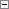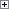Retrieves the length of a BigText variable.

Length := BigText.LENGTHProperty Value/Return Value

Type: Integer

The length of the BigText variable.Remarks

To delete the content in a BigText variable use the CLEAR Function. The syntax for the CLEAR function is shown in the following code snippet: CLEAR(BigText).Example

The following example demonstrates how to retrieve the length of a BigText variable. This example requires that you create the following variables and text constant in the C/AL Globals window.

Variable name DataType

MyBigText

BigText

VarLength

Integer

Text constant name ENU value

Text000

VarLength = %1

In this example, the BigText variable is initialized with the text ‘ABCDEFG’. The length, which is 7, is stored in the VarLength variable and displayed in a message box.Copy Code